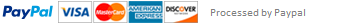### Java Homework: BingeWatch, DivisibleBy,LeapYear and AverageGrade classes

1.Using the provided classname and main method, write a program that prompts the user with the phrase "Enter Two Numbers: ", that then reads in two integers typed on the keyboard and outputs their sum, difference, and product, each on a different line. Your program should only output the values you compute, with no text or labels to identify them.

2. Using the provided classname and main method, write a program as follows: Elias had some extra time at home this summer and decided to binge watch some TV shows. To do so more efficiently, he asked you to write a program to calculate how many hours it will take to binge any given show. Elias will provide you with three numbers, in the following order: the number of seasons in the show, the number of episodes per season, and the length of each episode in minutes. Your program should read in these values without any prompts and output the phrase "This show will take X hours and Y minutes to watch in its entirety." with X and Y replaced with the appropriately-calculated values.

public class BingeWatch{

public static void main(String[] args){

}

}

3.

Using the provided classname and main method, write a program in which the input is a number entered by the user and the output is a space-separated string of the digits in the range [1,9] which that number is evenly divisible by. For example, if the user inputs "28", the output would be "1 2 4 7", because 28 is evenly divisible into 1, 2, 4, and 7.

public class DivisibleBy{

public static void main(String[] args){

}

}

4. Using the provided classname and main method, write a program that reads in a year entered by the user and determines if the entered year is a leap year. If it is, print "Yes". Otherwise, print nothing at all. You should only have an output if the year is a leap year.

(Hint: Every year that is exactly divisible by four is a leap year, except for years that are exactly divisible by 100, but these centurial years are leap years if they are exactly divisible by 400. For example, the years 1700, 1800, and 1900 are not leap years, but the years 1600 and 2000 are. Source.)

public class LeapYear{

public static void main(String[] args){

}

}

5. Using the provided classname and main method, write a program that reads in test scores line-by-line entered by the user until a "-1" is entered. When all numbers have been read in, the program should print out the highest score, the average score (as an integer), and the number of tests taken in this order on separate lines. Assume that there will always be at least one valid score preceeding the "-1", and that all inputs are integer values.

(Test scores should only be integer values from the range [0-100], but the user can enter whatever integers they want. All invalid inptuts should be disregarded by your program.)

public static void main(String[] args){

}

}

6. Using the provided classname and main method, write a program that reads in a positive integer number entered by the user and outputs a space-separated list consisting of that number, followed by all odd positive integers less than it in descending order.

public class Countdown{

public static void main(String[] args){

}

}

7. Write a program that reads in a positive integer entered by the user and outputs all positive integers less than or equal to that number that are perfect squares, each on its own line. For example, if the user entered "72", the output would be:

1

4

9

16

25

36

49

64

public class PerfectSquare {

public static void main(String[] arg) {

}

}

8. Using the provided classname, write a program that reads in an integer number. The number will tell you how many strings you should read in. The program will then read in that many strings. When the program is stopped, it should output the number of strings that fall alphabetically in the range a-m, a space, and the number of strings that alphabetically fall in the range n-z, based on the first letter of each string, ignoring the "stop".

For example, if the input consisted of:

4

apple

banana

carrot

zebra

You are being told to read in four strings. Your program should read in each of these strings, process them, then out outuput "3 1", because three of the words are in the range a-m, and one of the words is in the range n-z.

public class CountStrings{

public static void main(String[] args){

}

}

This assignment has been answered 2 times in private sessions.